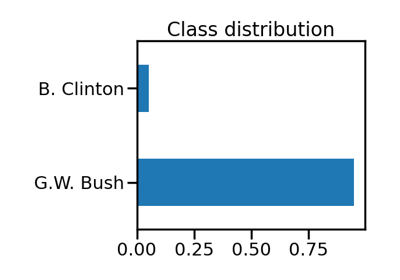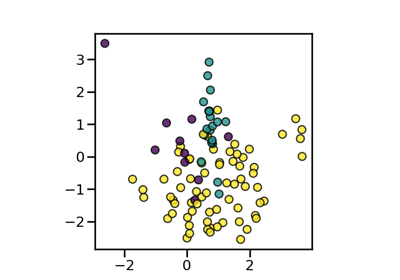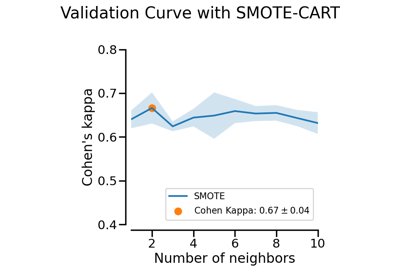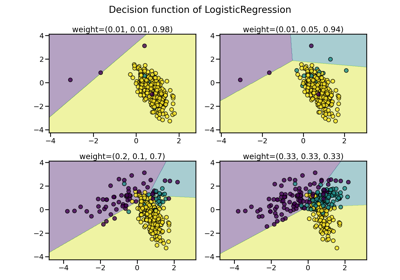# SMOTE¶

class imblearn.over_sampling.SMOTE(*, sampling_strategy='auto', random_state=None, k_neighbors=5, n_jobs=None)[source]

Class to perform over-sampling using SMOTE.

This object is an implementation of SMOTE - Synthetic Minority Over-sampling Technique as presented in .

Read more in the User Guide.

Parameters
sampling_strategyfloat, str, dict or callable, default=’auto’

Sampling information to resample the data set.

• When float, it corresponds to the desired ratio of the number of samples in the minority class over the number of samples in the majority class after resampling. Therefore, the ratio is expressed aswhereis the number of samples in the minority class after resampling andis the number of samples in the majority class.

Warning

float is only available for binary classification. An error is raised for multi-class classification.

• When str, specify the class targeted by the resampling. The number of samples in the different classes will be equalized. Possible choices are:

'minority': resample only the minority class;

'not minority': resample all classes but the minority class;

'not majority': resample all classes but the majority class;

'all': resample all classes;

'auto': equivalent to 'not majority'.

• When dict, the keys correspond to the targeted classes. The values correspond to the desired number of samples for each targeted class.

• When callable, function taking y and returns a dict. The keys correspond to the targeted classes. The values correspond to the desired number of samples for each class.

random_stateint, RandomState instance, default=None

Control the randomization of the algorithm.

• If int, random_state is the seed used by the random number generator;

• If RandomState instance, random_state is the random number generator;

• If None, the random number generator is the RandomState instance used by np.random.

k_neighborsint or object, default=5

If int, number of nearest neighbours to used to construct synthetic samples. If object, an estimator that inherits from KNeighborsMixin that will be used to find the k_neighbors.

n_jobsint, default=None

Number of CPU cores used during the cross-validation loop. None means 1 unless in a joblib.parallel_backend context. -1 means using all processors. See Glossary for more details.

SMOTENC

Over-sample using SMOTE for continuous and categorical features.

SMOTEN

Over-sample using the SMOTE variant specifically for categorical features only.

BorderlineSMOTE

Over-sample using the borderline-SMOTE variant.

SVMSMOTE

Over-sample using the SVM-SMOTE variant.

ADASYN

KMeansSMOTE

Over-sample applying a clustering before to oversample using SMOTE.

Notes

See the original papers:  for more details.

Supports multi-class resampling. A one-vs.-rest scheme is used as originally proposed in .

References

1(1,2,3)

N. V. Chawla, K. W. Bowyer, L. O.Hall, W. P. Kegelmeyer, “SMOTE: synthetic minority over-sampling technique,” Journal of artificial intelligence research, 321-357, 2002.

Examples

>>> from collections import Counter
>>> from sklearn.datasets import make_classification
>>> from imblearn.over_sampling import SMOTE
>>> X, y = make_classification(n_classes=2, class_sep=2,
... weights=[0.1, 0.9], n_informative=3, n_redundant=1, flip_y=0,
... n_features=20, n_clusters_per_class=1, n_samples=1000, random_state=10)
>>> print('Original dataset shape %s' % Counter(y))
Original dataset shape Counter({1: 900, 0: 100})
>>> sm = SMOTE(random_state=42)
>>> X_res, y_res = sm.fit_resample(X, y)
>>> print('Resampled dataset shape %s' % Counter(y_res))
Resampled dataset shape Counter({0: 900, 1: 900})


Methods

 fit(X, y) Check inputs and statistics of the sampler. fit_resample(X, y) Resample the dataset. get_params([deep]) Get parameters for this estimator. set_params(**params) Set the parameters of this estimator.
fit(X, y)[source]

Check inputs and statistics of the sampler.

You should use fit_resample in all cases.

Parameters
X{array-like, dataframe, sparse matrix} of shape (n_samples, n_features)

Data array.

yarray-like of shape (n_samples,)

Target array.

Returns
selfobject

Return the instance itself.

fit_resample(X, y)[source]

Resample the dataset.

Parameters
X{array-like, dataframe, sparse matrix} of shape (n_samples, n_features)

Matrix containing the data which have to be sampled.

yarray-like of shape (n_samples,)

Corresponding label for each sample in X.

Returns
X_resampled{array-like, dataframe, sparse matrix} of shape (n_samples_new, n_features)

The array containing the resampled data.

y_resampledarray-like of shape (n_samples_new,)

The corresponding label of X_resampled.

get_params(deep=True)[source]

Get parameters for this estimator.

Parameters
deepbool, default=True

If True, will return the parameters for this estimator and contained subobjects that are estimators.

Returns
paramsdict

Parameter names mapped to their values.

set_params(**params)[source]

Set the parameters of this estimator.

The method works on simple estimators as well as on nested objects (such as Pipeline). The latter have parameters of the form <component>__<parameter> so that it’s possible to update each component of a nested object.

Parameters
**paramsdict

Estimator parameters.

Returns
selfestimator instance

Estimator instance.

## Examples using imblearn.over_sampling.SMOTE¶